# How to Change Shape of Comment Box in Excel

We often add comments in excel to record some tips, add some additional message or anything else. The comment box is a square with light yellow background by default. Actually, the shape of comment box can be changed in excel. We can change the default shape of comment box by some other shapes imbedded in Change Shape function. This article will show you the method to change the shape by what you want.

Add a comment in current worksheet for cell B2.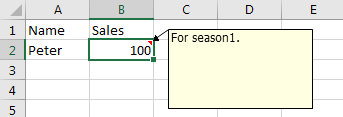Check current functions for comment. Obviously, there is no function for changing the shape of comment box.So, we need to change the shape by other ways.

## Change Shape of Comment Box by Change Shape Function

Change Shape function is hidden in excel quick access toolbar. As pre-condition we should made it visible in excel.

Step 1: Click File->Options to enter Excel Options window.

Step 2: Click Quick Access Toolbar in the left panel, then select ‘Drawing Tools|Format Tab’ in ‘Choose commands from’ dropdown list.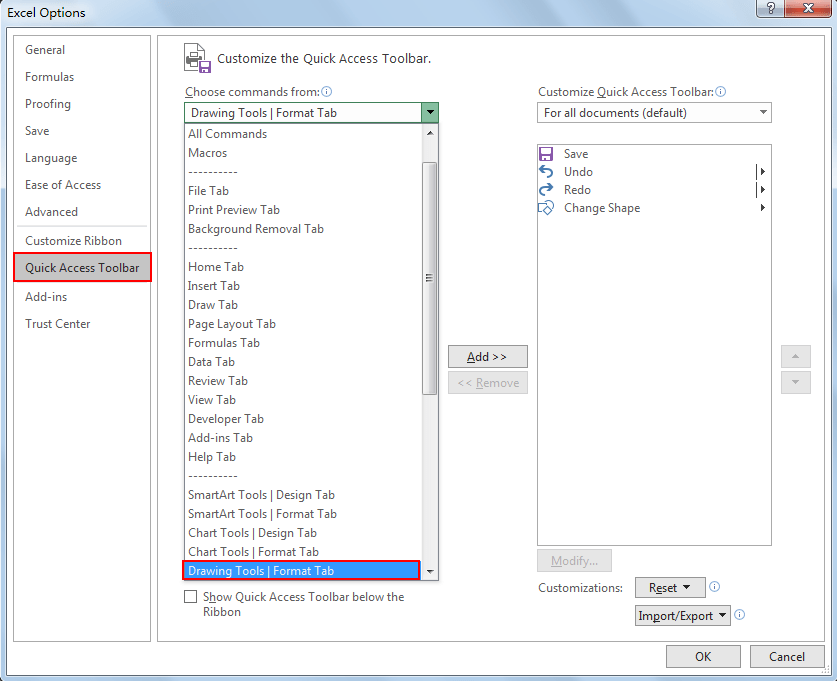Step 3: In ‘Drawing Tools|Format Tab’, select ‘Change Shape’, then click Add to move it to Quick Access Toolbar.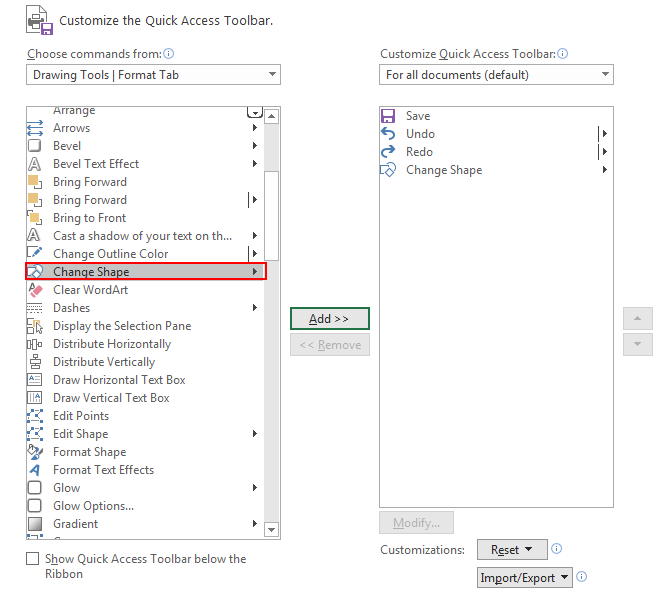Step 4: Then click OK to confirm settings and quit Excel Options. Verify that Change Shape icon is displayed in Quick Access Toolbar now.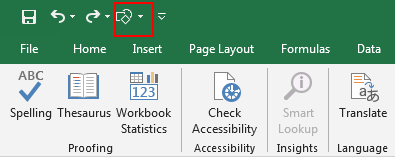After above preparation, we can change the shape of comment box by the new adding Change Shape function now.

Step 5: Click on Review->Edit Comment. Verify that the comment box is shown. But the Change Shape icon is disabled.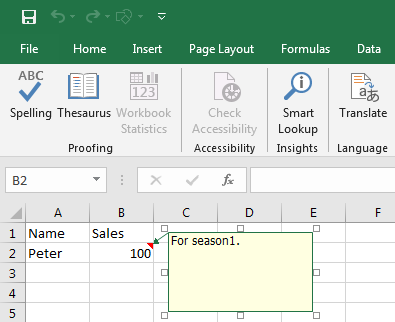Step 6: Click on the small square around the comment box border. This time Change Shape function is enabled.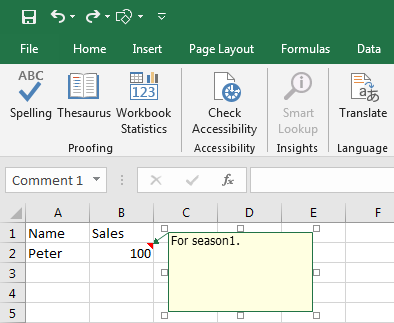Step 7: Click on the Change Shape icon to load all shapes.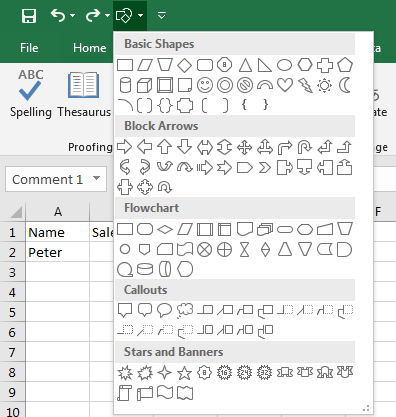Step 8: Select a shape you want to change. Then verify that the comment box is changed to the shape we selected properly.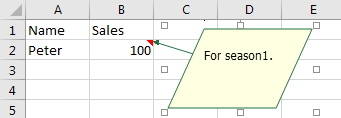Notes:

1. This shape is only applied for the selected comment box. It is not applied for all existing comments. If you want change shape of all comments, you have to change shape one by one refer to above steps.
2. The comment’s text cannot adjust to the selected shape automatically. See example below: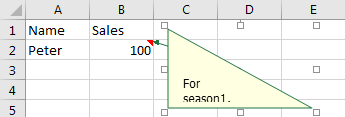Related Posts

Calculate Number of Hours between Two Times

Calculating the difference between two times might be a valuable statistic for subsequent computations or averages, whether you're producing a time sheet for staff or recording personal exercises. While Excel has a plethora of complex functions, including date and time ...

Calculate Loan Interest in Given Year

When you borrow money, you are supposed to repay it gradually. Lenders, on the other hand, want to be compensated for their services and the risk they incur by lending you money. That is, you will not just repay the ...

Calculate Interest Rate for Loan

The interest rate is the fee charged by a lender to a borrower and is expressed as a percentage of the principal—the lent amount. The interest rate on a loan is often expressed as an annual percentage rate, abbreviated as ...

Calculate Interest for Given Period

Using the IPMT function in Excel, we can compute the interest payment on any loan. This step-by-step tutorial will guide Excel users of all skill levels through the process to calculate interest for given period. Finally, the formula: =IPMT(B3/12,1,B5,-B2) The ...

How To Use Excel GCD Function

This post will guide you how to use Excel GCD function with syntax and examples in Microsoft excel. Description The Excel GCD function Returns the greatest common divisor of two or more integers. So you can use the GCD function ...

Calculate A Ratio From Two Numbers In Excel

In elementary mathematics, a ratio is a connection or comparison between two or more integers. For example, ratios are often expressed as ":" to demonstrate the relationship between two numbers. You would think that manually calculating a ratio from two ...

How To Use Excel RRI Function

This post will guide you how to use Excel RRI function with syntax and examples in Microsoft excel. Description The Excel RRI function Returns an equivalent interest rate for the growth of an investment. So you can use the RRI ...

CAGR Formula Examples in Excel

CAGR in Excel is a formula that calculates the compound annual growth rate for any invested amount over the specified years or timeframe. Although there is no direct function in Excel that can help us identify the CAGR value, there ...

Build Hyperlink With VLOOKUP in Excel

You might have come across a task in which you were assigned to build hyperlinks, which seems very easy, and if you are new to excel or don't have enough experience with it, then you might wonder about doing this ...

Break ties with helper COUNTIF and column

Suppose you got a task to adjust the values that contain the ties; what would be your first attempt to break the ties of the given value? If you are wondering about doing this task manually, let me add that ...

Sidebar